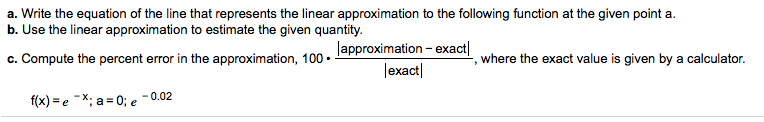a. Write the equation of the line that represents the linear approximation to the following function at the given point a.b. Use the linear approximation to estimate the given quantity.C. Compute the percent error in the approximation, 100 .where the exact value is given by a calculator.exact-0.02f(x)-e-x; a = 0:

Questionhelp_outlineImage Transcriptionclosea. Write the equation of the line that represents the linear approximation to the following function at the given point a. b. Use the linear approximation to estimate the given quantity. C. Compute the percent error in the approximation, 100 . where the exact value is given by a calculator. exact -0.02 f(x)-e-x; a = 0: fullscreen
Step 1

The expression for calculating linear approximation is shown on board. We substitute the values of a, f(a), f'(a) to get the required equation of line.

Step 2

To find e^-0.02 , we substitute the value x=0.02 in the expression of linear  approximation.

Step 3

The calculation for % errro...

Want to see the full answer?

See Solution

Want to see this answer and more?

Our solutions are written by experts, many with advanced degrees, and available 24/7

See Solution
Tagged in

Calculus